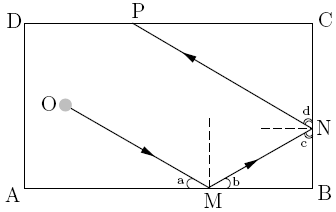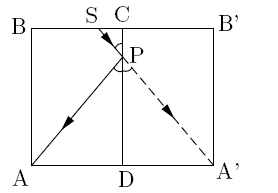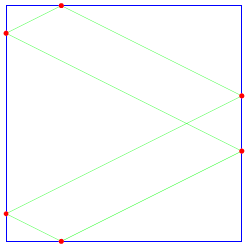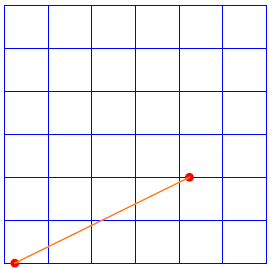MATH EXPLORERS' CLUB Cornell Department of Mathematics

# Billiards and Puzzles

 Introduction1. Rectangular Billiards 2. Circular/Elliptical Tables 3. Triangular Billiards 4. Puzzles 5. Two-Hole Billiards References## Rectangular Billiards

The game of billiards on a rectangular table is very popular and it has rather simple mathematical rules. In order to describe these rule, consider the rectangular table ABCD depicted below and assume that the gay ball hits the cushion AB at point M. Then it will bounce from this cushion, hitting the BC cushion at another point N, then bouncing again to point P on CD and so on. If we assume that there is no friction with the table then the ball will go on forever, unless it eventually hit one of the corners of the table. The only important rule is how the ball bounces from the border: the angle a is equal to angle b, both shown in the figure below. In other words, the incoming angle is equal to the outgoing angle.In the above picture, angles a and b are equal and so are angles c and d. The path that the ball describes in its movement is called the trajectory of a billiard ball. Although the rules are simple, the rectangular table produces interesting and surprising problems and puzzles as we will see in Lessons #4 and #5. Notice also that the same set of rules apply for all convex polygonal tables, instead of rectangular ones. In particular they apply for equilateral triangles, thus yielding powerful and complicated problems and puzzles as we will see in Lecture #3. An important property of billiards is unfolding the billiard table. This concept is again simple. Consider the rectangle ABCD and reflect it symmetrically along the CD border, thus producing two more vertices A' and B' and two rectangles as shown in the figure.Suppose that we have a ball at an arbitrary point S on the BC side and we wish to determine a point P on the CD side so that the ball bounces from the cushion at P and hits the pocket located at A (suppose there are pockets at every corner). This can be quite tedious if we don't unfold the billiard table. Suppose however that we do unfold it as shown in the figure above. Then in order to find point P we simply need to intersect line SA' with CD. This is because in order to describe the trajectory in the rectangle ABCD, we continue it in the rectangle CDB'A', with the undertanding that hitting pocket A' would be the same as hitting pocket A. One can easily see that the set of rules are clearly satisfied as angles A'PD and APD are the same.

Exercise #1: Unfold the billiard table ABCD across all sides and draw a picture. Perform this at least 10 times until you obtain a reasonable tesselation. Find 3 points, each on a different side of the rectangle ABCD, such that the ball at S enters pocket C.

Consider now a square billiard table. One would like to know if it is possible to hit a ball located at a given point on the lower boundary in such a way that it returns to the same point after exactly 6 bounces, as shown below. This question, as long as the pictures are from Reference #7.In order to answer such questions, it is easier to simply unfold the billiard table, thus making a finite grid in the first quadrant of the plane. The two red points in the picture are in fact the same point, regarded inside the initial table.We draw a line joining these two points and that is the trajectory of the ball regarded in the unfolded billiard table. Notice that it crosses exactly 5 borders, thus dividing the line segment into exactly 6 pieces. This means that the ball bounces exactly 6 times before returning to the original point.

Exercise #2: Redo the problem but with 10 bounces instead of 6.
Remark: It is possible in fact to shoot the ball in such a way that the trajectory comes arbitrarily close to any point on the boundary, i.e. the trajectory is dense. This happens when the angle at which we shoot the ball is an irrational multiple of π.

 Top Next lesson